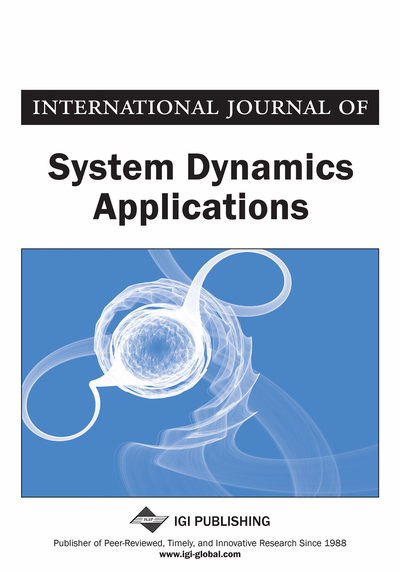# A Novel Quota Sampling Algorithm for Generating Representative Random Samples given Small Sample Size

Ahmed M. Fouad (Department of Computer Engineering, Cairo University, Cairo, Giza, Egypt), Mohamed Saleh (Department of Operations Research and Decision Support, Cairo University, Cairo, Giza, Egypt) and Amir F. Atiya (Department of Computer Engineering, Cairo University, Cairo, Giza, Egypt)
Copyright: © 2013 |Pages: 17
DOI: 10.4018/ijsda.2013010105
OnDemand PDF Download:
Available
\$37.50
No Current Special Offers

## Abstract

In this paper, a novel algorithm is proposed for sampling from discrete probability distributions using the probability proportional to size sampling method, which is a special case of Quota sampling method. The motivation for this study is to devise an efficient sampling algorithm that can be used in stochastic optimization problems -- when there is a need to minimize the sample size. Several experiments have been conducted to compare the proposed algorithm with two widely used sample generation methods, the Monte Carlo using inverse transform, and quasi-Monte Carlo algorithms. The proposed algorithm gave better accuracy than these methods, and in terms of time complexity it is nearly of the same order.
Article Preview
Top

## 2. Problem Definition

The problem is to generate random samples from a discrete probability distribution, P(x),that are selected to be as representative as possible, given the constraint of small sample size, N. This situation arises in stochastic optimization problems; where there is a need to generate too many sequences of the samples. For example, such sequences (of the samples)can be used to represent a random variable in a stochastic simulation model, which is embedded in the fitness function of a genetic algorithm. In such situations, computational time considerations will limit the sample size. The generated random samples must have two important properties: fitting the required probability distribution and independence (i.e. each random sample must be an independent sample drawn from the defined distribution). To check whether these desirable properties have been achieved, a number of tests can be performed. The tests can be placed into two categories, according to the properties of interest: goodness of fit, and independence.

Failure to reject the null hypothesis means that the evidence of lack of fit has not been detected by this test. The Kolmogorov – Smirnov test compares the CDF, F(x), of the required distribution with the empirical CDF, SN(x), of the generated samples. It is based on calculating the largest absolute deviation between the actual CDF and the empirical CDF. Therefore, the test is based on the statistic:

D = max |F(x) – SN(x)| (2)

The sampling distribution of D is known as a function of N.

• Autocorrelation test: Ljung-Box test(Cryer & Chan, 2008; Ljung & Box, 1978)is used to test the overall randomness between the generated samples, where the hypotheses are as follows:H0: xi~ independently (Null hypothesis) (3) H1: xi !~ independently

Failure to reject the null hypothesis means that the evidence of dependence has not been detected by this test. The Ljung-Box test is based on the test statistic:

(4) where:

## Complete Article List

Search this Journal:
Reset
Volume 11: 2 Issues (2022): 1 Released, 1 Forthcoming
Volume 10: 4 Issues (2021)
Volume 9: 4 Issues (2020)
Volume 8: 4 Issues (2019)
Volume 7: 4 Issues (2018)
Volume 6: 4 Issues (2017)
Volume 5: 4 Issues (2016)
Volume 4: 4 Issues (2015)
Volume 3: 4 Issues (2014)
Volume 2: 4 Issues (2013)
Volume 1: 4 Issues (2012)
View Complete Journal Contents Listing